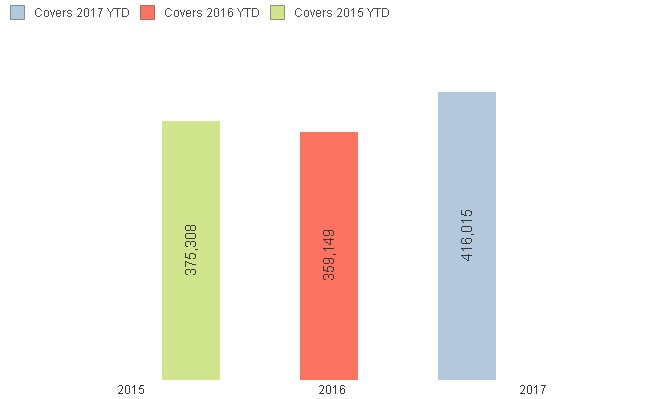# QlikView App Development

Discussion Board for collaboration related to QlikView App Development.

Announcements
Leverage your QlikView investment to modernize BI – see how! Join Group
cancel
Showing results for
Did you mean:
HighlightedContributor III

## Multiple Dates within Expression

Hi

Is it possible to create an expression that contains the equivalent of an OR statement

SQL would be;

select sum(sales)

Where (Yearfield = 2017 AND Datefield <= '2017/05/10')

OR (Yearfield = 2016 AND Datefield <= '2016/05/10')

OR (Yearfield = 2015 AND Datefield <= '2015/05/10')

So I would end up with numbers for YTD 3 year comparison.

I want to use this to calculate an average so I can see how the average plots year on year after the same period.

Tags (4)
1 Solution

Accepted Solutions
HighlightedContributor III

## Re: Multiple Dates within Expression

I have been able to find the solution '+' is OR

For;

(Cover=1 AND Year = Current )

OR (Cover=1 AND Year = Previous AND date <= today last year)

OR (Cover=1 AND Year = 2 yrs ago AND date <= today 2 yrs ago)

Expression =;

=sum({<[Cover]={'1'},[Financial Year]={"\$(v.Date.Current.Year)"}>

+<[Cover]={'1'} ,[Financial Year]={"\$(v.Date.Year.Prior)"},[Transaction Date]={"<=\$(v.Date.Date.TodayLastYear)"}>

+<[Cover]={'1'} ,[Financial Year]={"\$(v.Date.Year.Prior2)"},[Transaction Date]={"<=\$(v.Date.Date.Today2YearsAgo)"}>

}[Quantity Sold])

4 Replies
HighlightedAnonymous
Not applicable

## Re: Multiple Dates within Expression

you could try

...

where yeartodate(Datefield,0) or yeartodate(Datefield,-1) or yeartodate(Datefield,-2) ...

You could also do this in a loop in combination with concatenate

HighlightedSpecialist

## Re: Multiple Dates within Expression

Hi, John!

Would it be usefull?

=sum(if(Yearfield = 2017 AND Datefield <= '2017/05/10',sales,0))+

sum(if(Yearfield = 2016 AND Datefield <= '2016/05/10',sales,0))+

sum(if(Yearfield = 2015 AND Datefield <= '2015/05/10',sales,0))

HighlightedContributor III

## Re: Multiple Dates within Expression

Hi,

Thanks for your replies, unfortunately neither resolved my issue

I've tried another example below;

=sum({<[Cover]={'1'}

AND (

([Financial Year]={"\$(v.Date.Current.Year)"},[Transaction Date]={"<=\$(v.Date.Yesterday)"})

OR ([Financial Year]={"\$(v.Date.Year.Prior)"},[Transaction Date]={"<=\$(v.Date.Date.TodayLastYear)"})

OR ([Financial Year]={"\$(v.Date.Year.Prior2)"},[Transaction Date]={"<=\$(v.Date.Date.Today2YearsAgo)"})

)

>}[Quantity Sold])

This would produce a simple chart as in the example below however the example below has the 3 date ranges as separate expressions, I need all 3 as 1 expression as I need to over lay a line graph, which is currently resulting in 3 separate dots rather than a single trend line.HighlightedContributor III

## Re: Multiple Dates within Expression

I have been able to find the solution '+' is OR

For;

(Cover=1 AND Year = Current )

OR (Cover=1 AND Year = Previous AND date <= today last year)

OR (Cover=1 AND Year = 2 yrs ago AND date <= today 2 yrs ago)

Expression =;

=sum({<[Cover]={'1'},[Financial Year]={"\$(v.Date.Current.Year)"}>

+<[Cover]={'1'} ,[Financial Year]={"\$(v.Date.Year.Prior)"},[Transaction Date]={"<=\$(v.Date.Date.TodayLastYear)"}>

+<[Cover]={'1'} ,[Financial Year]={"\$(v.Date.Year.Prior2)"},[Transaction Date]={"<=\$(v.Date.Date.Today2YearsAgo)"}>

}[Quantity Sold])